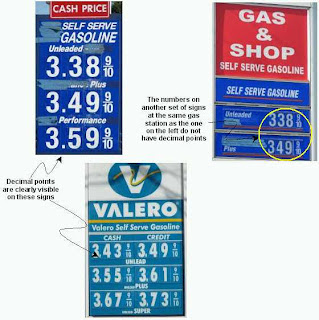## Sunday, May 27, 2007

### Strange Numbers, Mixing Decimal Fractions with Ordinary Fractions

Although such number mix-ups are not common, I have seen them several times on gas stations. Finally I took some photos.A few observations:

• I suspect that, if a student wrote an answer to a math problem in this form, the teacher will not accept it as valid.
• My son pointed out that these numbers are like having a second decimal point, such as 3.38.9, which is wrong.
• The values that these numbers represent are obviously clear; adding the decimal point, if noticed at all, causes no confusion: The ordinary fraction stands for 9/10 of a cent, not of a dollar.
• This can be viewed to be an example of mixed bases in the place-value columns, which is very acceptable and common practice but requires special notation. For example, the time 7:49:25.83 is correct and unambiguous. The different place values are separated by colons and a decimal point. The leftmost place value is either 12- or 24-base and may consist of a single digit or two; the next two place values are based 60 and the rightmost one is a decimal fraction of the preceding one (seconds), separated from it by a decimal point and, in this example, contains two decimal place values.
• The prices on the pumps at these gas stations are completely decimal, such as \$3.389 and \$3.499.

Does the inclusion of the decimal point add any more information? Does it serve any purpose? What did the people who placed the decimal points with the fractions tried to accomplish? Or is it the result of lack of understanding of how to express numerical values? (I did not ask any station attendant about their signs.)I am not questioning the presence of the 9/10 fractions because having them is a well-established practice in retail-gasoline pricing.

Question. Has anyone seen similar mixing of decimal fractions with ordinary fraction anywhere other than on gas-stations' price signs?